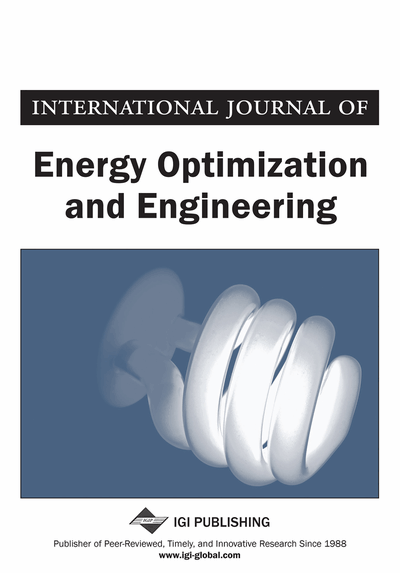# The Use of the Data Transformation Techniques in Estimating the Shape Parameter of the Weibull Distribution for the Wind Speed

Yeliz Mert Kantar (Department of Statistics, Faculty of Science, Anadolu University, Eskisehir, Turkey) and Ibrahim Arik (Department of Statistics, Faculty of Science, Anadolu University, Eskisehir, Turkey)
DOI: 10.4018/ijeoe.2014070102
Available
\$37.50
No Current Special Offers

## Abstract

In recent years, the Weibull distribution has been commonly used and recommended to model the wind speed. Therefore, many estimators have been proposed to find the best method to estimate the parameters of the Weibull distribution. Particularly, the estimator based on regression procedures with the Weibull probability plot are often used because of its computational simplicity and graphical presentation. However, when the procedure is applied, in many cases heteroscedasticity or non-normality of the error terms may be encountered. One way to handle this problem is using transformation techniques. In this study, the regression estimation based on data transformation is considered to estimate the parameters of the Weibull distribution. The simulation results show that the considered estimator based on the data transformation for the shape parameter of the Weibull distribution provides better performance than least squares estimator in terms of bias and mean square errors for the most of the considered cases.
Article Preview
Top

## Introduction

It is known that the Weibull distribution is an accepted lifetime model in reliability (Abernethy, 1996) and also it is the most widely-used distribution to estimate wind energy potential (Akdag & Dinler, 2009; Ani et al. 2012; Badmasti et al.2012; Saleh et al. 2012; Bagiorgas et al. 2011). For this reason, in the literature, many estimators have been proposed for the parameters of the Weibull distribution and these estimators have been compared according to different criteria (Gibbons & Vance, 1981; Bergman, 1986; Zixing, 1993; Tiryakioğlu & Hudak, 2007; Drapella & Kosznik, 1999; Hossain & Zimmer, 2003; Kantar & Senoglu, 2008; Hung, 2001; Hossain & Howlader, 1996; Lu et al. 2004; Marks, 2005; Zhang et al. 2008; Zyl, 2012). Particularly, regression procedures with the Weibull probability plot (WPP) are often used for estimating the parameters of the Weibull distribution (Tiryakioglu & Hudak, 2007; Drapella & Kosznik, 1999; Hossain & Zimmer, 2003; Hung, 2001; Hossain & Howlader, 1996; Lu et al. 2004; Marks, 2005; Zhang et al. 2008) because of its computational simplicity and graphical presentation. However, when the procedure is applied, in many cases both non-constant variance (heteroscedasticity) and asymmetry of the error terms may be encountered (Zyl, 2012; Boudt et al. 2011). When these cases occur, the least squares (LS), which is a well-known estimator with probability plot, loses its desirable properties (Kantar & Senoglu, 2008) and thus, the LS procedure does not apply for obtaining estimates. One of the practical approaches to deal with these cases is the data transformations, which are used extensively to obtain constant variance (homoscedasticity) and symmetry of errors (Box & Cox, 1982; Carrol & Ruppert, 1984; Carrol & Welsh, 1988; Collins, 1991; Sakia, 1992; Manly, 1976; Ruppert & Aldershof, 1989; Williams, 1997).

In this study, the regression estimation based on transformations with the WPP is proposed to estimate the parameters of the Weibull distribution. We consider the Manly’s transformation instead of the well-known Box-Cox, which allows negative data values under a condition of its shifted version. The simulation results show that the considered estimator for the shape parameter of the Weibull distribution provides better performance than the LS estimator and the Hung’s weighted least squares (WLS) estimator in terms of bias and mean square errors for the most of the considered sample sizes and the shape parameter cases.

The rest of this paper is organized as follows: Section 2 provides the description of the Weibull distribution and the regression procedures with the WPP. Section 3 introduces data transformation and the formulation of the Manly’s transformation and also provides regression estimation after transformation. Alternative regression estimation for the Weibull distribution is briefly reminded in Section 4. To show the performance of the estimator based on the transformation, a simulation study is presented in Section 5. The considered estimators for the Weibull distribution is discussed on wind speed data in Section 6 and finally, the last section summarizes the conclusions of the study.

## Complete Article List

Search this Journal:
Reset
Volume 11: 4 Issues (2022): 1 Released, 3 Forthcoming
Volume 10: 4 Issues (2021)
Volume 9: 4 Issues (2020)
Volume 8: 4 Issues (2019)
Volume 7: 4 Issues (2018)
Volume 6: 4 Issues (2017)
Volume 5: 4 Issues (2016)
Volume 4: 4 Issues (2015)
Volume 3: 4 Issues (2014)
Volume 2: 4 Issues (2013)
Volume 1: 4 Issues (2012)
View Complete Journal Contents Listing